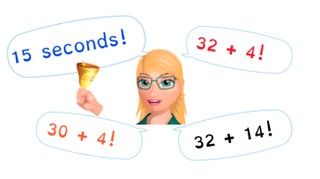Learn to quickly and correctly solve addition problems to 100.

No account needed.8,000 schools use Gynzy92,000 teachers use Gynzy1,600,000 students use Gynzy

## General

Students learn to solve addition problems to 100 quickly and correctly.

## Standards

CCSS.Math.Content.2.NBT.B.5

## Learning objective

Students will be able to quickly and correctly solve addition problems up to 100 in my head.

## Introduction

Practice two addition problems as a class. Use the visual support. One problem contains a one digit number added to a two digit number.

## Instruction

Explain to students what automaticity is, and what automaticity in addition problems means. Tell students that they are going to practice solving addition problems in their head within a given time. The goal is to solve the addition problem within 5, 4, and even 3 seconds using the classroom strategy. Start by discussing the problems out loud using the classroom strategy, the first in 5 seconds, then 4, and finally in 3 seconds. Then ask students to solve the problems within those times and discuss the answers. Tell students that have trouble solving within time that this is something that improves with practice. Then challenge the class to solve three problems in 15 seconds and four problems within 20 seconds.

Check that students are able to quickly and correctly solve addition problems to 100 by asking the following questions:
- Why is it useful to be able to quickly solve addition problems?
- How do you solve an addition problem if you only have 20 seconds to find the answer?

## Quiz

Students practice with sets of addition problems and are challenged to complete them in progressively less time.

## Closing

Ask students to solve five addition problems in 25 seconds. There are sound fragments with addition problems. Next play addition speedway. First make groups of 7 or 5. Split these groups into 2 and set one student in the middle and have them come up with addition problems. The group or side that has the correct answer first gets a point. The student who gets the answer correct moves to the middle to come up with the next problem. Play until your reach 3 points.

## Teaching tips

Students who have difficulty with this learning goal can be supported by asking students to solve addition problems to 100 without a time limit. You then can ask students to solve problems with a time limit of 30 seconds, 25 seconds, 20 seconds, etc.

### The online teaching platform for interactive whiteboards and displays in schools

• Save time building lessons

• Manage the classroom more efficiently

• Increase student engagement